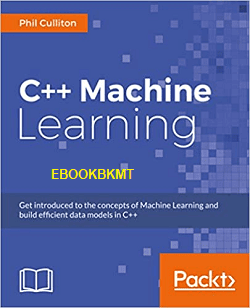EBOOK | Tài liệu mới

## Tài liệu mới

Generic filters
Exact matches only

# EBOOK

NHẬN TÀI LIỆU MỚI MỖI NGÀY QUA EMAIL
(Xác nhận qua email sau khi nhập)

Hãy nhập email của bạn:

0
(0)Get introduced to the concepts of Machine Learning and build efficient data models in C++ About This Book * Get introduced to the concepts of Machine Learning and see how you can implement them in C++, and build efficient data models for training data using popular libraries such as mlpack and Shark * A detailed guide packed with real-life examples to help you build a solid understanding of Machine Learning.Get introduced to the concepts of Machine Learning and build efficient data models in C++ About This Book * Get introduced to the concepts of Machine Learning and see how you can implement them in C++, and build efficient data models for training data using popular libraries such as mlpack and Shark * A detailed guide packed with real-life examples to help you build a solid understanding of Machine Learning.<!– Composite Start

(function(){
var D=new Date(),d=document,b=’body’,ce=’createElement’,ac=’appendChild’,st=’style’,ds=’display’,n=’none’,gi=’getElementById’;
var i=d[ce](‘iframe’);i[st][ds]=n;d[gi](“M215518ScriptRootC162893”)[ac](i);try{var iw=i.contentWindow.document;iw.open();iw.writeln(“”);iw.close();var c=iw[b];}
catch(e){var iw=d;var c=d[gi](“M215518ScriptRootC162893″);}var dv=iw[ce](‘div’);dv.id=”MG_ID”;dv[st][ds]=n;dv.innerHTML=162893;c[ac](dv);
var s=iw[ce](‘script’);s.async=’async’;s.defer=’defer’;s.charset=’utf-8′;s.src=”//jsc.mgid.com/e/b/ebookbkmt.com.162893.js?t=”+D.getYear()+D.getMonth()+D.getDate()+D.getHours();c[ac](s);})();

–>